Courses

# Test: Sheet Metal Operations - 1

## 20 Questions MCQ Test RRB JE for Mechanical Engineering | Test: Sheet Metal Operations - 1

Description
This mock test of Test: Sheet Metal Operations - 1 for Mechanical Engineering helps you for every Mechanical Engineering entrance exam. This contains 20 Multiple Choice Questions for Mechanical Engineering Test: Sheet Metal Operations - 1 (mcq) to study with solutions a complete question bank. The solved questions answers in this Test: Sheet Metal Operations - 1 quiz give you a good mix of easy questions and tough questions. Mechanical Engineering students definitely take this Test: Sheet Metal Operations - 1 exercise for a better result in the exam. You can find other Test: Sheet Metal Operations - 1 extra questions, long questions & short questions for Mechanical Engineering on EduRev as well by searching above.
QUESTION: 1

Solution:
QUESTION: 2

### A blank of 30 mm diameter is to be produced out of 10 mm thick sheet on a simple die. If 6%clearance is recommended, then the nominal diameters of pie and punch are respectively

Solution:

It is blanking operation so clearance must be provided on punch.
Therefore, Die size = blank size = 30 mm
Punch size = blank size – 2C = 30 -2 x 0.06 x t = 30 – 2 x 0.06 x 10 = 28.8 mm

QUESTION: 3

### In sheet metal blanking, shear is provided on punches and dies so that

Solution:

Shear is provided on punch and dies to reduce press load.

QUESTION: 4

Match the correct combination for following metal working processes.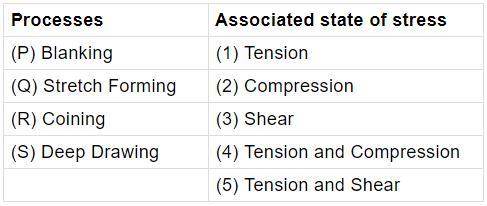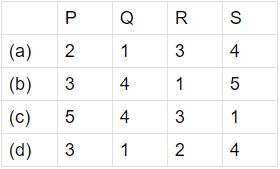Solution:
QUESTION: 5

A metal disc of 20 mm diameter is to be punched from a sheet of 2 mm thickness. The punch and the die clearance is 3%. The required punch diameter is

Solution:

It is blanking operation
Therefore Diameter of die = metal disc diameter = 20 mm
3% clearance (c) = 0.06 mm on both side of the die (of sheet thickness)
Therefore Diameter of punch = 20 – 2c = 20 – 2 x 0.06 = 19.88 mm

QUESTION: 6

10 mm diameter holes are to be punched in a steel sheet of 3 mm thickness. Shear strength of the material is 400 N / mm2 and penetration is 40%. Shear provided on the punch is 2 mm.The blanking force during the operation will be

Solution:

The blanking force (P) =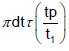= π x  10 x  3 x 400 x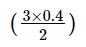= 22 .62kN

QUESTION: 7

Match List-I (metal forming process) with List-II (Associated feature) and select the correct answer using the codes given below the Lists: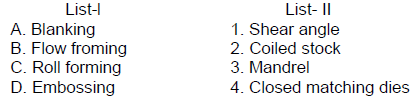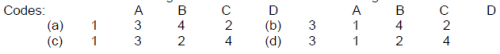Solution:
QUESTION: 8

In a blanking operation to produce steel washer, the maximum punch load used in 2 x 105 N.The plate thickness is 4 mm and percentage penetration is 25. The work done during this shearing operation is

Solution: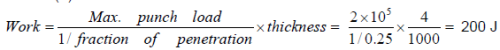QUESTION: 9

A 50 mm diameter disc is to be punched out from a carbon steel sheet 1.0 mm thick. The diameter of the punch should be

Solution:

explanation :  using formula,
The diameter of punch = diameter of disc - 2 × clearness

here it is given that ,

diameter of disc = 50mm

and clearance = 3% of disc thickness

= 3% of 1mm [ as thickness of disc = 1mm]

= 3 × 1/100 = 0.03 mm

so, The diameter of punch = 50mm - 2 × 0.03mm

= 50mm - 0.06mm

= 49.94 mm

hence, The diameter of punch should be 49.94 mm.

QUESTION: 10

Worm working or semi-hot working process, which is the plastic deformation of metal or alloy under conditions of temperature and strain rate, is performed to eliminate drawbacks of

Solution:
QUESTION: 11

Match List I (Operation) with List II (Explanation) and select the correct answer using the
codes given below the Lists: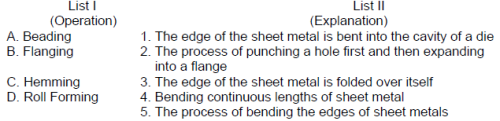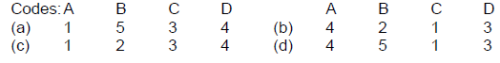Solution:
QUESTION: 12

In deciding the clearance between punch and die in press work in shearing, the following rule is helpful:

Solution:

In punching usable part is sheet so punch size is correct and clearance on die. In blanking usable part is punched out circular part so die size is correct and clearance on punch

QUESTION: 13

Consider the following steps in forging a connecting rod from the bar stock:
1. Blocking
2. Trimming
3. Finishing
4. Edging

Select the correct sequence of these operations using the codes given below:

Codes:

Solution:
QUESTION: 14

A hole is to be punched in a 15 mm thick plate having ultimate shear strength of 3N-mm-2. If the allowable crushing stress in the punch is 6 N-mm-2, the diameter of the smallest hole which can be punched is equal to

Solution: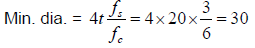QUESTION: 15

The order of radial clearance between the punch and die for shearing a steel plate of thickness ‘t’ (1 mm ≤2 mm) is

Solution:
QUESTION: 16

Which one of the following factor promotes the tendency for wrinking in the process of drawing?

Solution:
QUESTION: 17

The mode of deformation of the metal during spinning is

Solution:

Mode of deformation of metal during spinning is bending and stretching.

QUESTION: 18

Which one of the following is a continuous bending process in which opposing rolls are used to produce long sections of formed shapes from coil or strip stock?

Solution:
QUESTION: 19

A 1.5 mm thick sheet is subject to unequal biaxial stretching and the true strains in the directions of stretching are 0.05 and 0.09. The fh'1al thickness of the sheet in mm is

Solution:
QUESTION: 20

Consider the following statements:
Earring in a drawn cup can be due to non-uniform
1. speed of the press 2. clearance between tools
3. material properties 4. blank holding

Q. Which of these statements are correct?

Solution: